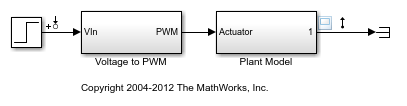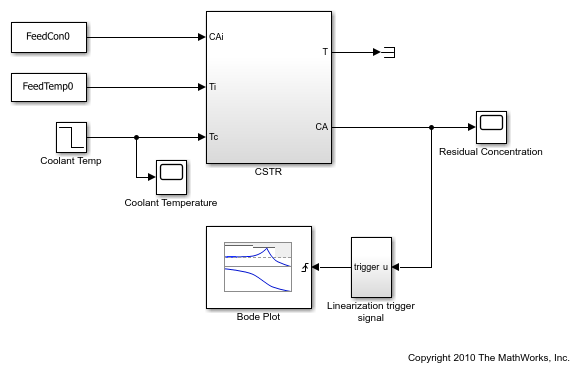# getlinio

Obtain linear analysis points from Simulink model, Linear Analysis Plots block, or Model Verification block

## Syntax

``io = getlinio(mdl)``
``io = getlinio(blockpath)``

## Description

example

````io = getlinio(mdl)` returns the analysis points defined in the Simulink® model `mdl`.```

example

````io = getlinio(blockpath)` returns the analysis points defined for the specified Linear Analysis Plots block or Model Verification block in a Simulink model.```

## Examples

collapse all

```mdl = 'scdpwm'; open_system(mdl) ```This model contains the following linear analysis points:

• Input perturbation at the output of the Step block

• Output measurement at the output of the Plant Model block

Obtain the analysis points from the model.

```io = getlinio(mdl) ```
```2x1 vector of Linearization IOs: -------------------------- 1. Linearization input perturbation located at the following signal: - Block: scdpwm/Step - Port: 1 2. Linearization output measurement located at the following signal: - Block: scdpwm/Plant Model - Port: 1 ```

You can use these analysis points for subsequent linearizations of the model using the `linearize` command or an `slLinearizer` interface.

```open_system('scdcstr') ```This model contains a Bode Plot block that is configured with the following linear analysis points:

• Input perturbation at the output of the Coolant Temp block

• Output measurement at the `CA` output of the CSTR block

Obtain the analysis points from the Bode Plot block.

```io = getlinio('scdcstr/Bode Plot') ```
```2x1 vector of Linearization IOs: -------------------------- 1. Linearization input perturbation located at the following signal: - Block: scdcstr/Coolant Temp - Port: 1 2. Linearization output measurement located at the following signal: - Block: scdcstr/CSTR - Port: 2 ```

## Input Arguments

collapse all

Simulink model name, specified as a character vector or string. The model must be in the current working folder or on the MATLAB® path.

If the model is not open or loaded into memory, `getlinio` loads the model into memory.

Linear Analysis Plots block or Model Verification block, specified as a character vector or string that contains its full block path. The model that contains the block must be in the current working folder or on the MATLAB path.

## Output Arguments

collapse all

Analysis point set, returned as a linearization I/O object or a vector of linearization I/O objects. Use `io` to specify linearization inputs, outputs, and loop openings when using the `linearize` command. For more information, see Specify Portion of Model to Linearize.

Each analysis point has the following properties:

PropertyDescription
`Active`

Flag indicating whether to use the analysis point for linearization, specified as one of the following:

• `'on'` — Use the analysis point for linearization. This value is the default option.

• `'off'` — Do not use the analysis point for linearization. Use this option if you have an existing set of analysis points and you want to linearize a model with a subset of these points.

`Block`

Full block path of the block with which the analysis point is associated, specified as a character vector.

`PortNumber`

Output port with which the analysis point is associated, specified as an integer.

`Type`

Analysis point type, specified as one of the following:

• `'input'` — Input perturbation

• `'output'` — Output measurement

• `'loopbreak'` — Loop break

• `'openinput'` — Open-loop input

• `'openoutput'` — Open-loop output

• `'looptransfer'` — Loop transfer

• `'sensitivity'` — Sensitivity

• `'compsensitivity'` — Complementary sensitivity

For more information on analysis point types, see Specify Portion of Model to Linearize.

`BusElement`

Bus element name with which the analysis point is associated, specified as a character vector or `''` if the analysis point is not a bus element.

`Description`

User-specified description of the analysis point, which you can set for convenience, specified as a character vector.

### Topics

Introduced before R2006a